# 2 D Motion and Vectors Chapter 3 Chapter

• Slides: 27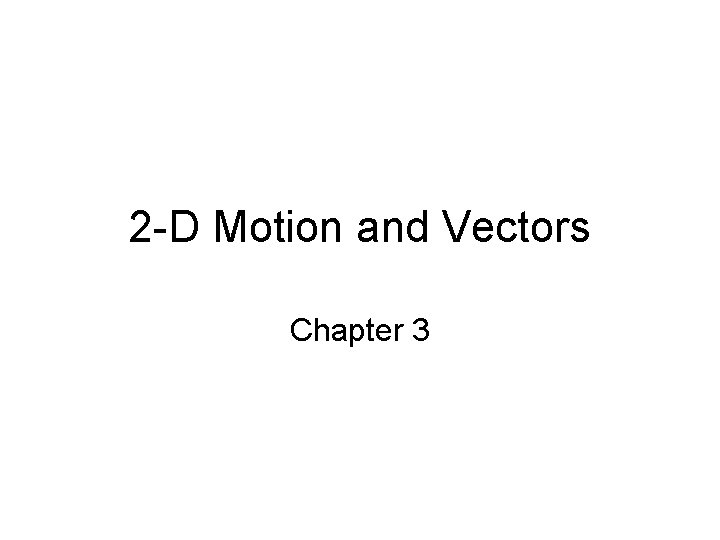2 -D Motion and Vectors Chapter 3Chapter 3 Review Questions • P 113… • 1 -13, 19, 22, 27, 30, 32, 34, 36, 40, 44, 47, 48, 50, 54, 60, 70.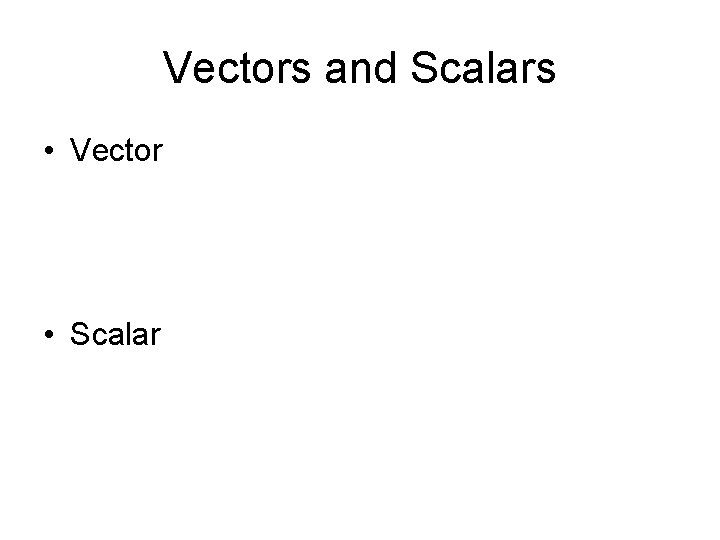Vectors and Scalars • Vector • ScalarQuestion(s) of the Day • A quantity that has no direction is a _______ quantity, while a quantity that has direction is a called a ____quantity.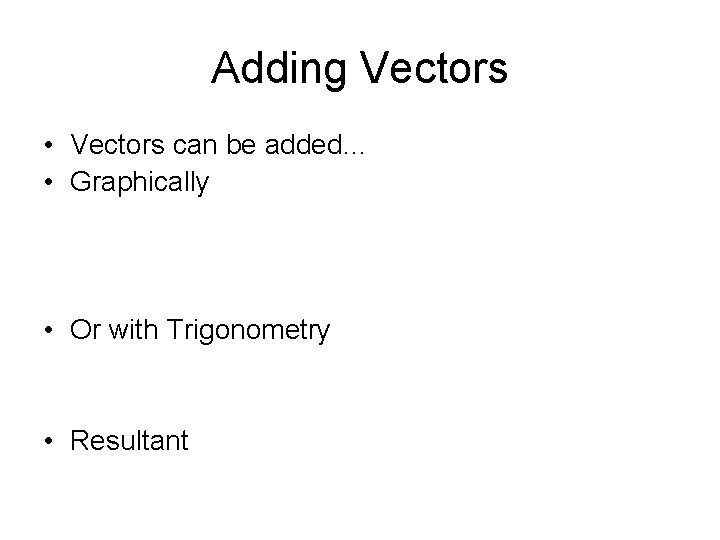Adding Vectors • Vectors can be added… • Graphically • Or with Trigonometry • ResultantTrigonometry • • What do you do if you injure your foot? Soh Cah Toa For right triangles…. Sine = Opposite/Hypotenuse Cosine = Adjacent/Hypotenuse Tangent = Opposite/Adjacent Pythagorean Theorem: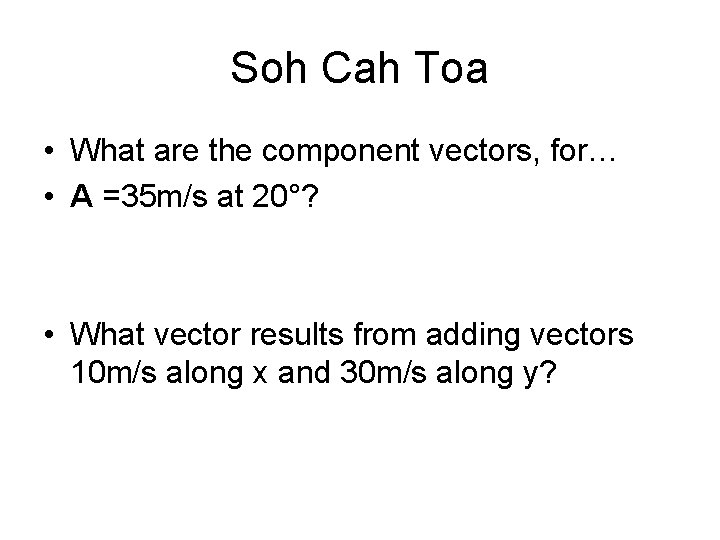Soh Cah Toa • What are the component vectors, for… • A =35 m/s at 20°? • What vector results from adding vectors 10 m/s along x and 30 m/s along y?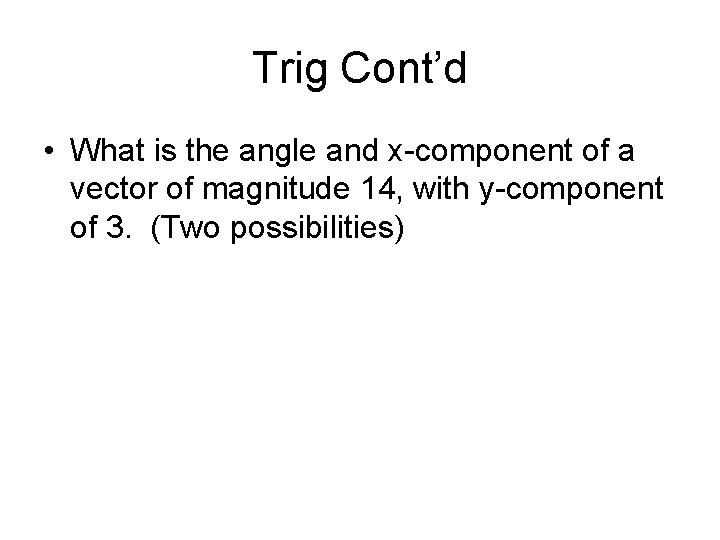Trig Cont’d • What is the angle and x-component of a vector of magnitude 14, with y-component of 3. (Two possibilities)Now adding with trigonometry • What is a + b if a = 13 at 20º and b = 16 at 120º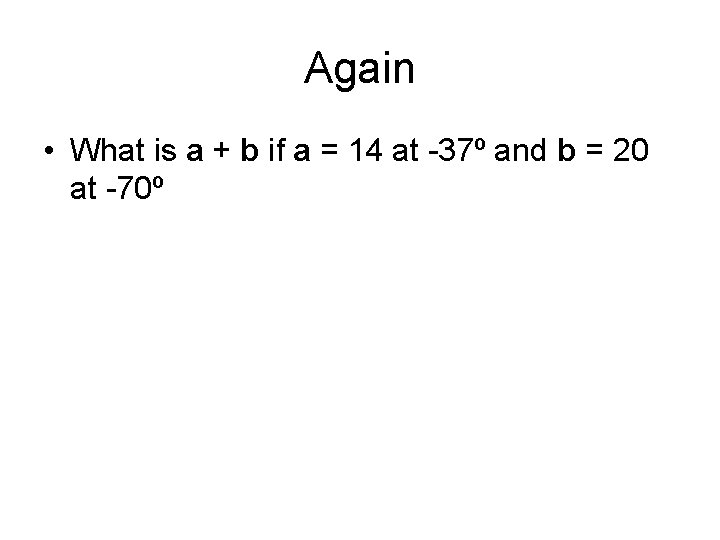Again • What is a + b if a = 14 at -37º and b = 20 at -70ºIn summary • Graphically, slide vectors (maintaining direction) add tip to tail • Trig: knowing one side and the angle, use trig functions to solve for other sides. • Trig: knowing sides, use inverse trig function to solve for angle. • Add x and y components, then use pythagorean theorem to get resultantQuestion(s) of the Day • Add graphically and by trigonometry: • 20 @ 30º + 40 @ 150º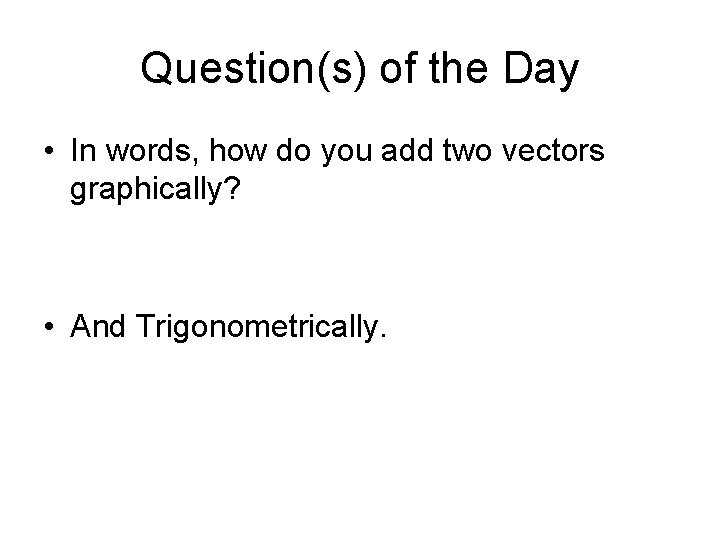Question(s) of the Day • In words, how do you add two vectors graphically? • And Trigonometrically.Other Vector Properties • Vectors can be moved parallel to themselves in a diagram • Vectors can be added in any order • To subtract a vector, add its opposite • vector(scalar) = vector • vector/scalar = vectorMotion in two dimensions • Motion can be analyzed one dimension at a time. • For projectiles, ignoring air resistance, • ay=-9. 8 m/s 2, ax =0 m/s 2 • All the constant acc eqns apply as before.launched horizontally • A ball is thrown from a 20 m cliff at 6 m/s horizontally. Where and when will it hit the ground?Question(s) of the Day • If an object is thrown with a velocity of 6 m/s at 45º, what will the x-component of it’s velocity be? • What range of values will the y-component of the velocity take during its flight if it is thrown from the ground and returns to the ground?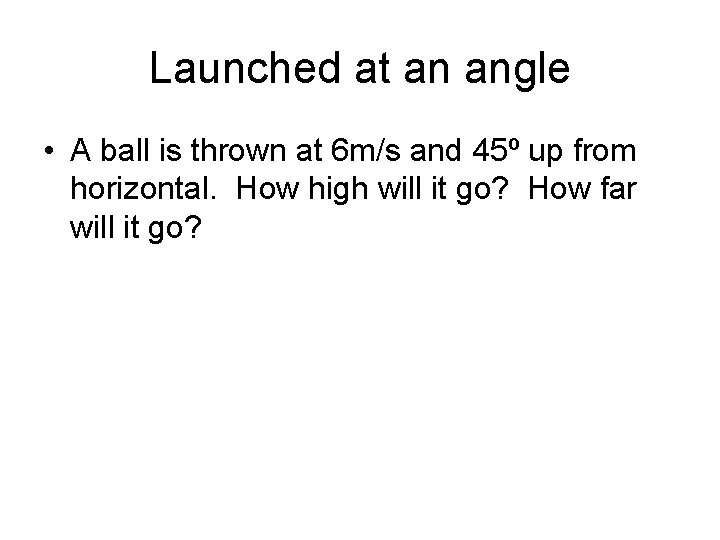Launched at an angle • A ball is thrown at 6 m/s and 45º up from horizontal. How high will it go? How far will it go?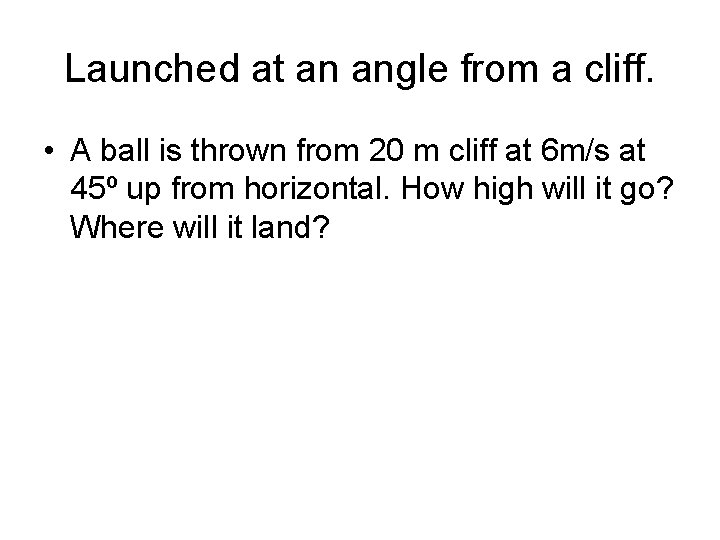Launched at an angle from a cliff. • A ball is thrown from 20 m cliff at 6 m/s at 45º up from horizontal. How high will it go? Where will it land?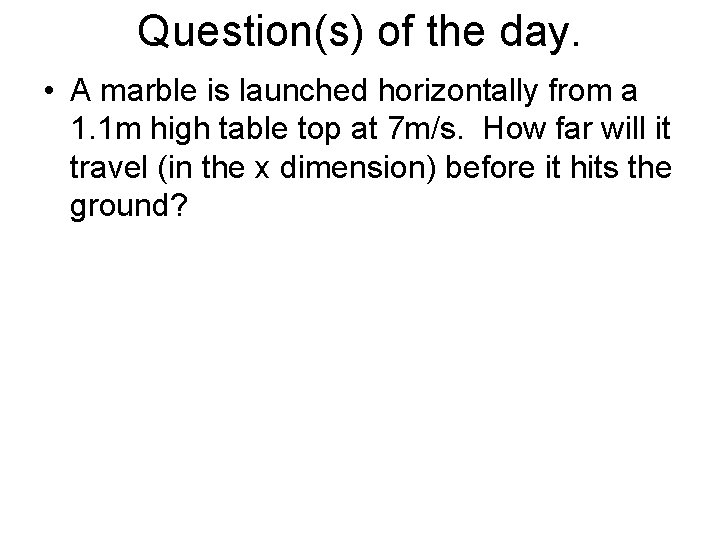Question(s) of the day. • A marble is launched horizontally from a 1. 1 m high table top at 7 m/s. How far will it travel (in the x dimension) before it hits the ground?A projectile follows a parabola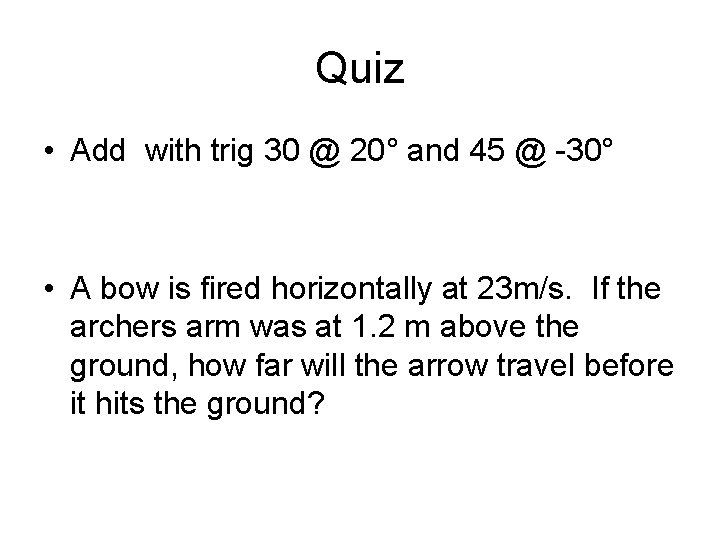Quiz • Add with trig 30 @ 20° and 45 @ -30° • A bow is fired horizontally at 23 m/s. If the archers arm was at 1. 2 m above the ground, how far will the arrow travel before it hits the ground?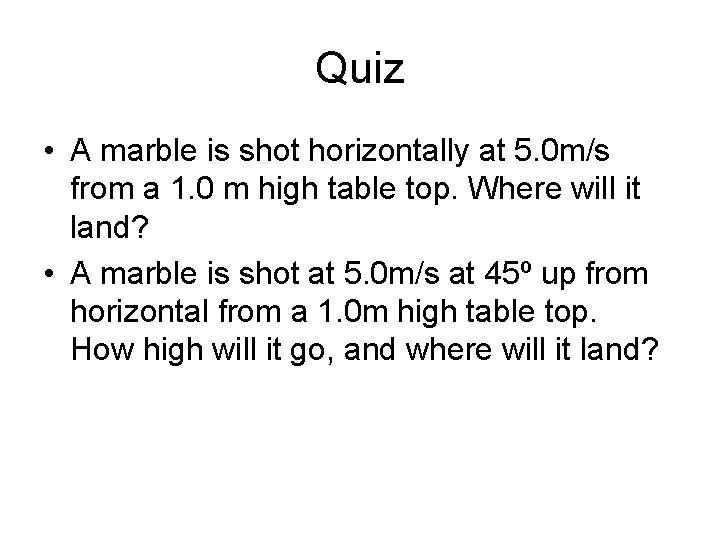Quiz • A marble is shot horizontally at 5. 0 m/s from a 1. 0 m high table top. Where will it land? • A marble is shot at 5. 0 m/s at 45º up from horizontal from a 1. 0 m high table top. How high will it go, and where will it land?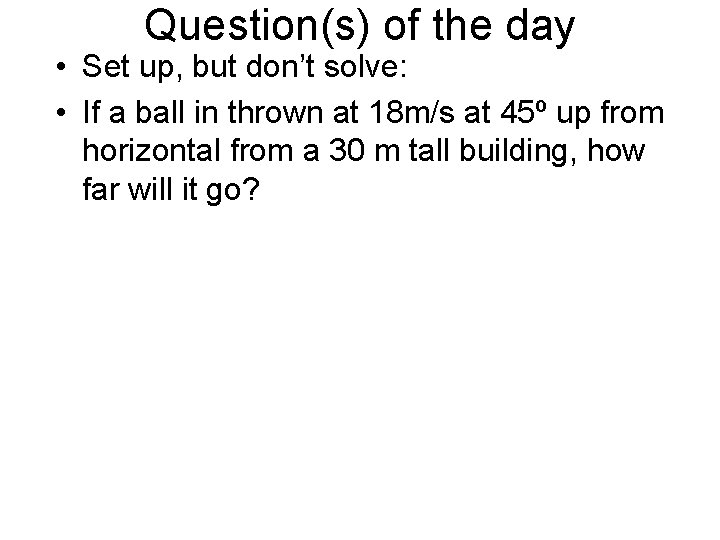Question(s) of the day • Set up, but don’t solve: • If a ball in thrown at 18 m/s at 45º up from horizontal from a 30 m tall building, how far will it go?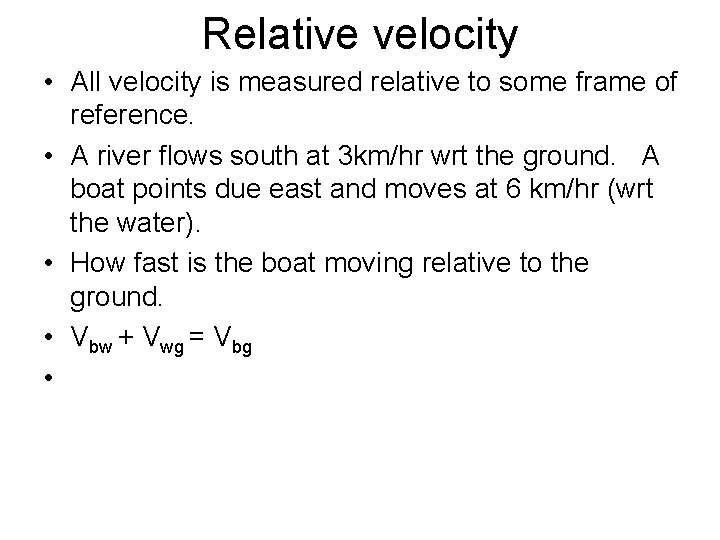Relative velocity • All velocity is measured relative to some frame of reference. • A river flows south at 3 km/hr wrt the ground. A boat points due east and moves at 6 km/hr (wrt the water). • How fast is the boat moving relative to the ground. • Vbw + Vwg = Vbg •another • I run at 5 mi/hr west wrt ground. • You run 5 mi/hr east wrt ground on same path. • What is my speed relative to yours? • Vmy + Vyg = Vmg • Vmy = Vmg –Vyg • Vmy = 5 – (-5) = 10 mi/hr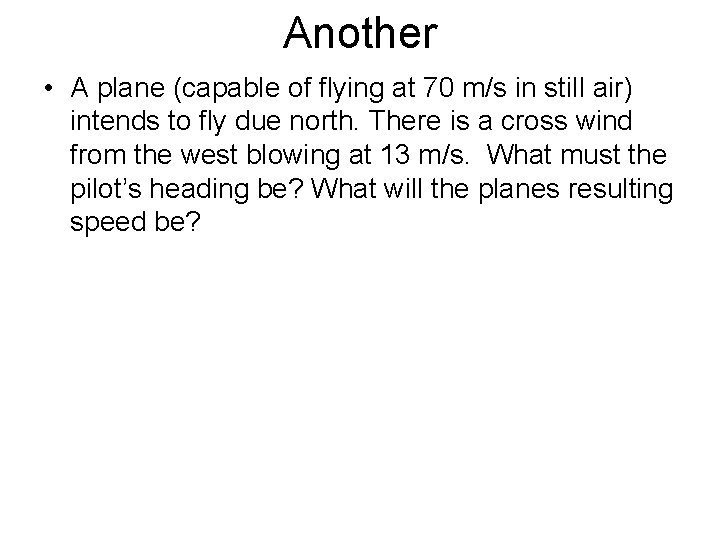Another • A plane (capable of flying at 70 m/s in still air) intends to fly due north. There is a cross wind from the west blowing at 13 m/s. What must the pilot’s heading be? What will the planes resulting speed be?